• 初等函数是由幂函数(power function)、指数函数(exponential function)、对数函数(logarithmicfunction)、三角函数(trigonometric function)、反三角函数(inverse trigonometic function)与常数经过有限次的有理运算...

初等函数是由幂函数(power function)、指数函数(exponential function)、对数函数(logarithmic function)、三角函数(trigonometric function)、反三角函数(inverse trigonometic function)与常数经过有限次的有理运算（加、减、乘、除、有理数次乘方、有理数次开方）及有限次函数复合所产生、并且能用一个解析式表示的函数。

转载于:https://www.cnblogs.com/jiangzhen/archive/2012/03/08/2385511.html
展开全文• ## 基本初等函数及图像

万次阅读 多人点赞 2019-11-06 14:47:58
本文介绍了基本初等函数的分类，基本初等函数的图像曲线 以及函数的基本性质


基本初等函数
基本初等函数的分类基本初等函数的图像函数的基本性质反函数

基本初等函数的分类
（1）常量函数 y=C (C为常数) （2）幂函数 y=xμ (μ∈R, μ≠0) （3）指数函数 y=ax (a>0, a≠1,特别当a=e时，记为 y=ex) （4）对数函数 y=logax (a>0, a≠1,特别当a=e时，记为 y=lnx) 这里对数函数y=logax是指数函数y=ax的反函数，y=lnx是 y=ex的反函数。 （5）三角函数 正弦函数 y=sin(x); 余弦函数 y=cos(x); 正切函数 y=tan(x)=sin(x)/cos(x); 余切函数 y=cot(x)=cos(x)/sin(x) （6）反三角函数 反正弦函数 y=arcsin(x) 反余弦函数 y=arccos(x) 反正切函数 y=arctan(x） 反余切函数 y=arccot(x)
基本初等函数的图像
1 常数函数 y=C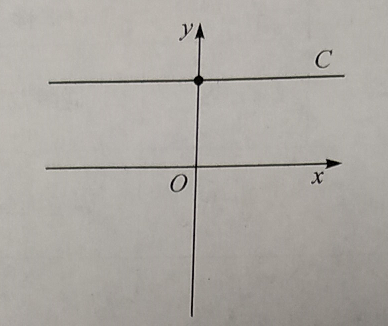2 幂函数 y=xμ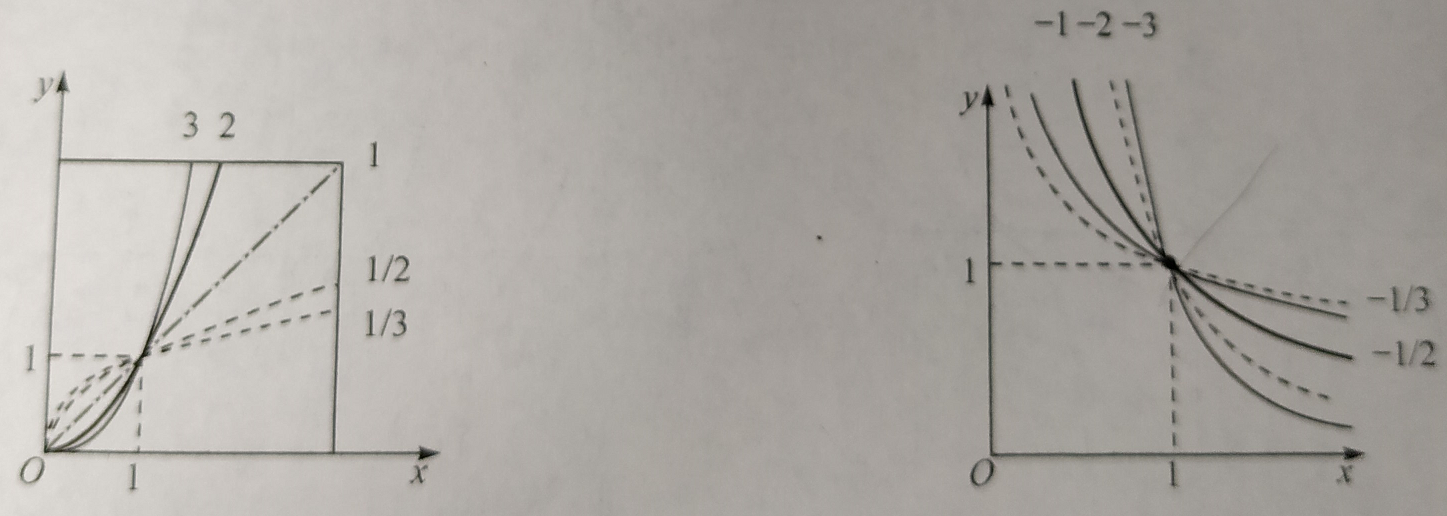3指数函数 y=ax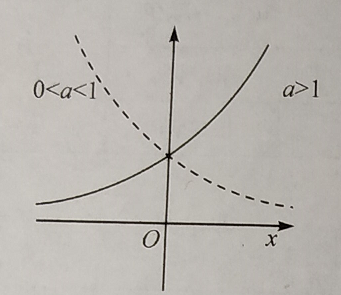4 对数函数 y=logax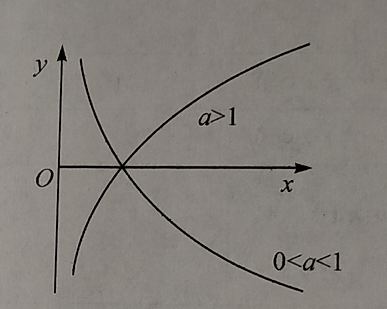5 正弦函数 y=sin(x)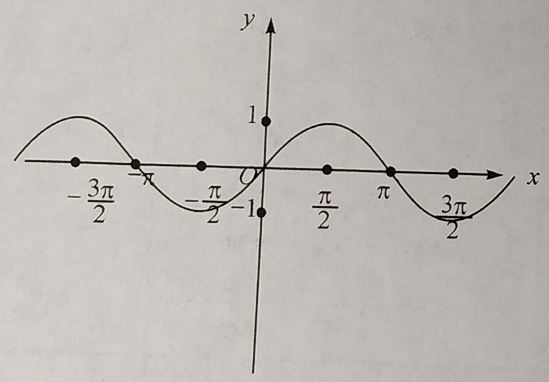6 余弦函数 y=cos(x)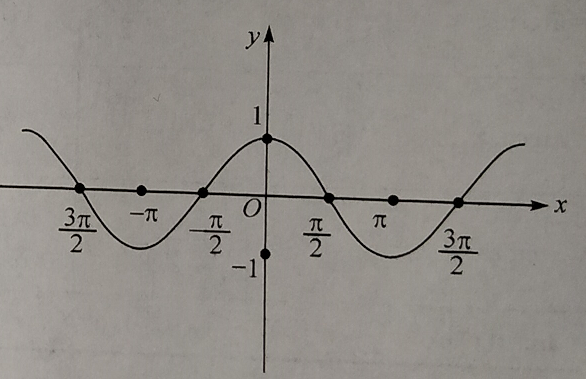7 正切函数 y=tan(x)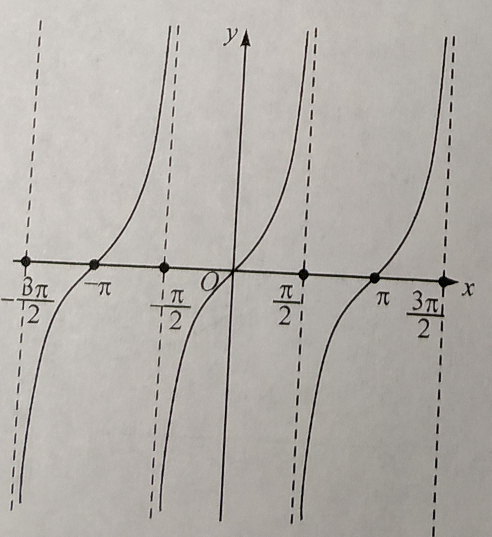8 余切函数 y=cot(x)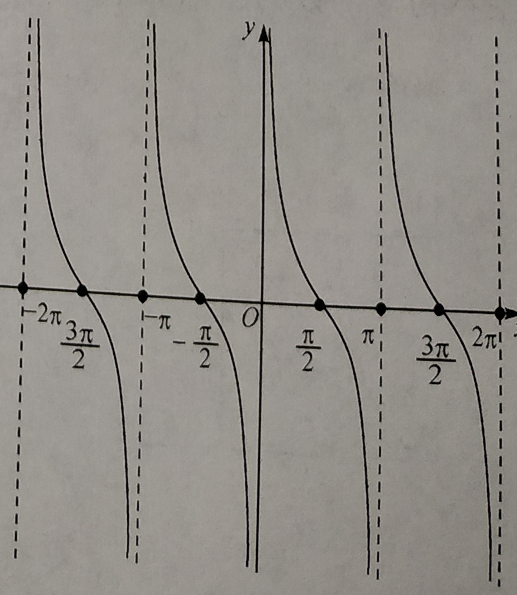9 反正弦函数 y=arcsin(x)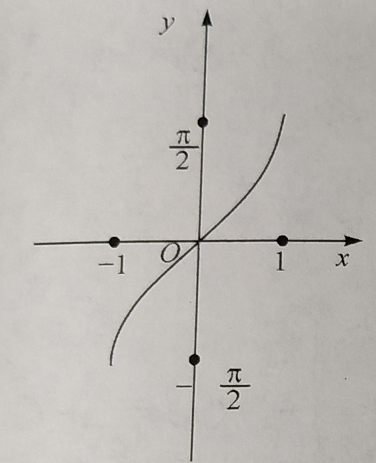10 反余弦函数 y=arccos(x)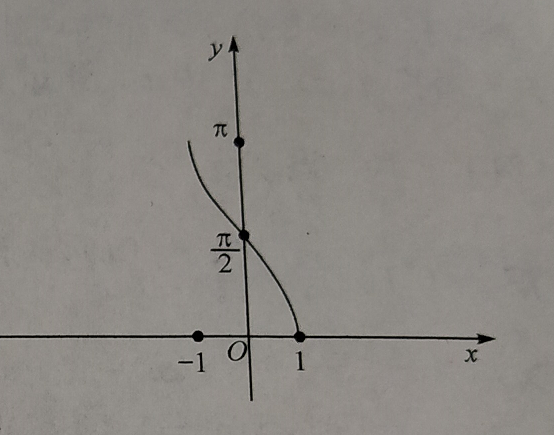11 反正切函数 y=arctan(x）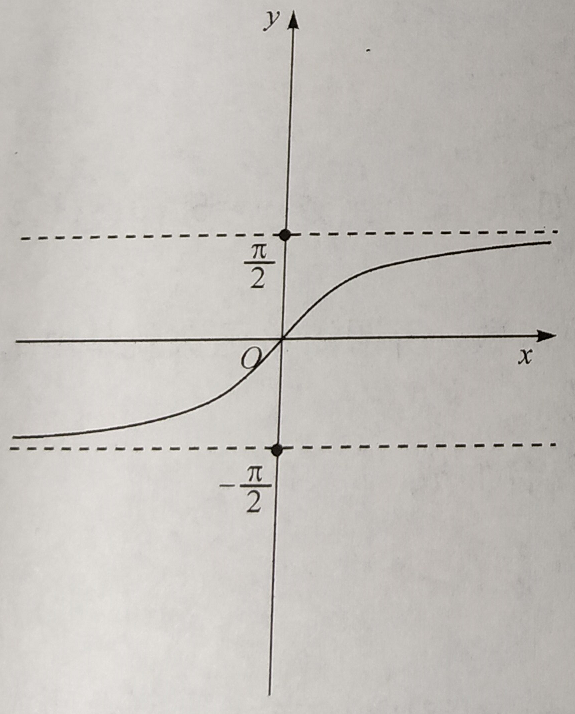12 反余切函数 y=arccot(x)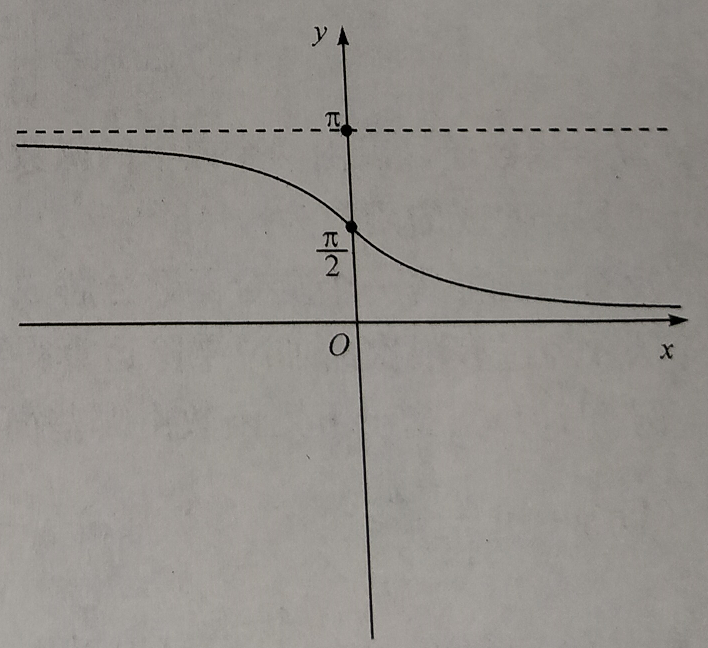函数的基本性质
1 有界性 2 单调性 设函数f(x)在D上有定义，如果对于D中任意两个数x1, x2, 当x1 < x2时，总有f(x1)<f(x2) (或 f(x1)>f(x2)), 则称f(x)在D上单调增加（或单调减少） 单调增加或单调减少的函数统称为单调函数。 3 奇偶性 设y=f(x), x∈D, 其中D关于原点对称，如果对于任意x∈D,总有 f(-x) = -f(x) (或 f(-x) = f(x))， 则称f(x)为奇函数（或偶函数）。 法则：两个偶函数的和是偶函数，两个奇函数的和是奇函数，两个偶函数的乘积是偶函数，两个奇函数的乘积也是偶函数，偶函数与奇函数的乘积是奇函数，偶函数与奇函数的和既不是偶函数也不是奇函数 4 周期性
反函数
展开全文函数曲线 函数的基本性质
• 初等函数是由基本初等函数经过有限次的四则运算和复合运算所得到的函数。基本初等函数初等函数在其定义区间内均为连续函数。高等数学将基本初等函数归为五类：幂函数、指数函数、对数函数、三角函数、...

初等函数是由基本初等函数经过有限次的四则运算和复合运算所得到的函数。基本初等函数和初等函数在其定义区间内均为连续函数。高等数学将基本初等函数归为五类：幂函数、指数函数、对数函数、三角函数、反三角函数。
比较头疼的是numpy中的幂函数不支持负数定义域，所以找了很多办法来解决该问题。
主函数代码如下：
#!/usr/bin/env python
# -*- coding: UTF-8 -*-
#                     _ooOoo_
#                   o8888888o
#                    88" . "88
#                 ( | -  _  - | )
#                     O\ = /O
#                 ____/---'\____
#                  .' \\| |// .
#                 / \\|||:|||// \
#               / _|||||-:- |||||- \
#                | | \\\ - /// | |
#              | \_| ''\---/'' | _/ |
#               \ .-\__ - ___/-. /
#            ___. .' /--.--\ . . __
#         ."" '< .___\_<|>_/___.' >'"".
#       | | : - \.;\  _ /;./ -  : | |
#          \ \ -. \_ __\ /__ _/ .- / /
#      ==-.____-.___\_____/___.-____.-'==
#                     =---='
'''
@Project ：pythonalgorithms
@File ：basicfunction.py
@Author ：不胜人生一场醉
@Date ：2021/7/19 17:39
'''
import matplotlib.pyplot as plt
import numpy as np
import math
import mpl_toolkits.axisartist as axisartist  # 导入坐标轴加工模块

# -----------------函数------------------
# 给定一个数集A，对A施加一个对应的法则/映射f，记做:f(A)，那么可以得到另外一个数集B，也就是可以认为B=f(A)；
# 那么这个关系就叫做函数关系式，简称函数。
# 三个重要的因素: 定义域A、值域B、对应的映射法则f。

if __name__ == "__main__":
# 一次函数
Linearfunction()
# 二次函数
# 幂函数
powerfunction()
# 指数函数
exponentialfunction()
# 对数函数
Logarithmicfunction()

一次函数代码如下：
# ---------------一次函数------------------
# 一次函数是函数中的一种，一般形如y=ax+b（a，b是常数，a≠0），其中x是自变量，y是因变量。
# 当b=0时，y=kx（k为常数，k≠0），y叫做x的正比例函数
# 当a=0时，y=b，则为常函数
def Linearfunction():
fig = plt.figure(figsize=(10, 8))
plt.rcParams['font.sans-serif'] = ['SimHei']  # 绘图中文
plt.rcParams['axes.unicode_minus'] = False  # 绘图负号
x = np.linspace(-10, 10, 100)
i = 1
alist = [-1, 0, 1]
blist = [-1, 0, 1]
for a in alist:
for b in blist:
plt.subplot(3, 3, i)
y = x * a + b
label = '{}*x+{}'.format(a, b)
plt.plot(x, y, label=label)
plt.legend()
i += 1
plt.show()

for a in alist:
for b in blist:
y = x * a + b
label = '{}*x+{}'.format(a, b)
plt.plot(x, y, label=label)
i += 1
plt.title("一次函数")
plt.legend()
plt.show()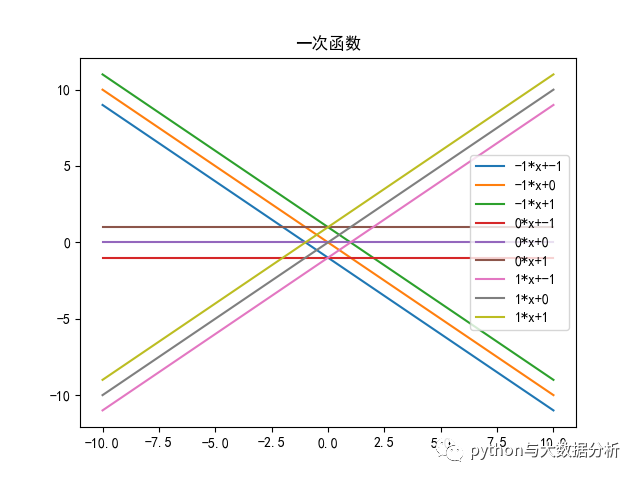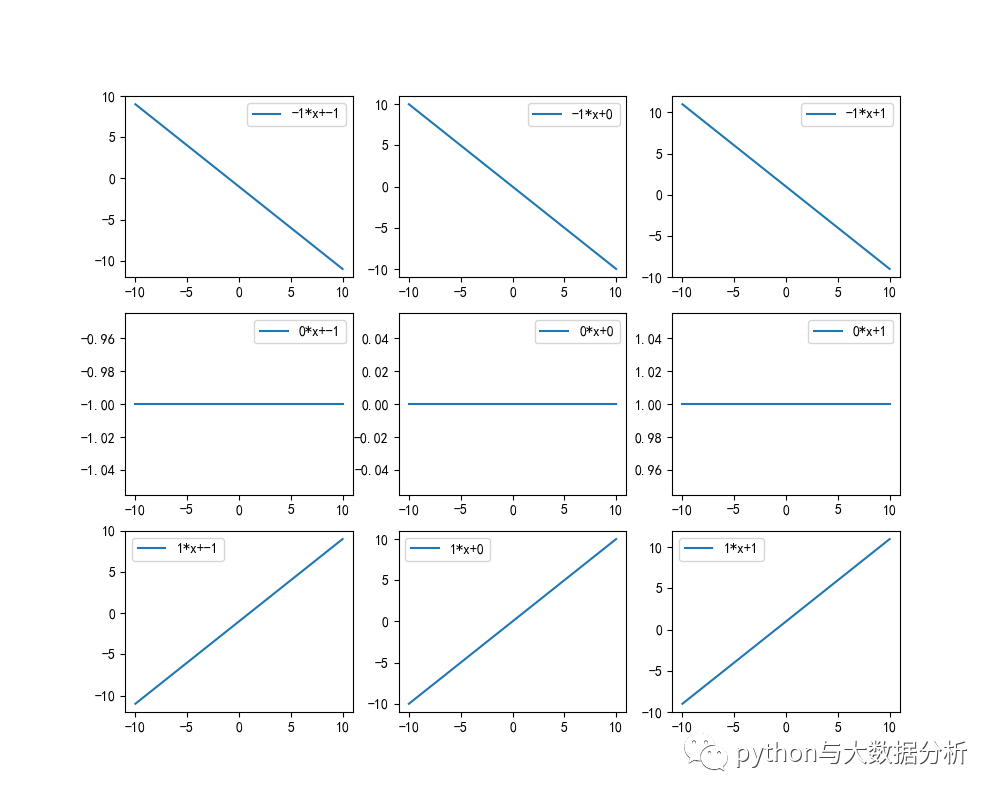二次函数代码如下：
# 二次函数（quadratic function）的基本表示形式为y=ax²+bx+c（a≠0）。
# 二次函数最高次必须为二次， 二次函数的图像是一条对称轴与y轴平行或重合于y轴的抛物线。
# 其中a称为二次项系数，b为一次项系数，c为常数项。x为自变量，y为因变量。等号右边自变量的最高次数是2。
# 如果令y值等于零，则可得一个二次方程。该方程的解称为方程的根或函数的零点。
# 顶点坐标 = (-b/2a,(4ac-b²)/4a
fig = plt.figure(figsize=(10, 8))
plt.rcParams['font.sans-serif'] = ['SimHei']  # 绘图中文
plt.rcParams['axes.unicode_minus'] = False  # 绘图负号
x = np.linspace(-2, 2, 100)

alist = [-1, 1]
blist = [-1, 0, 1]
clist = [-1, 0, 1]

for a in alist:
for b in blist:
for c in clist:
y = a * x * x + b * x + c
label = '{}*x*x+{}*x+{}'.format(a, b, c)
plt.plot(x, y, label=label)
plt.title("二次函数")
plt.legend()
plt.show()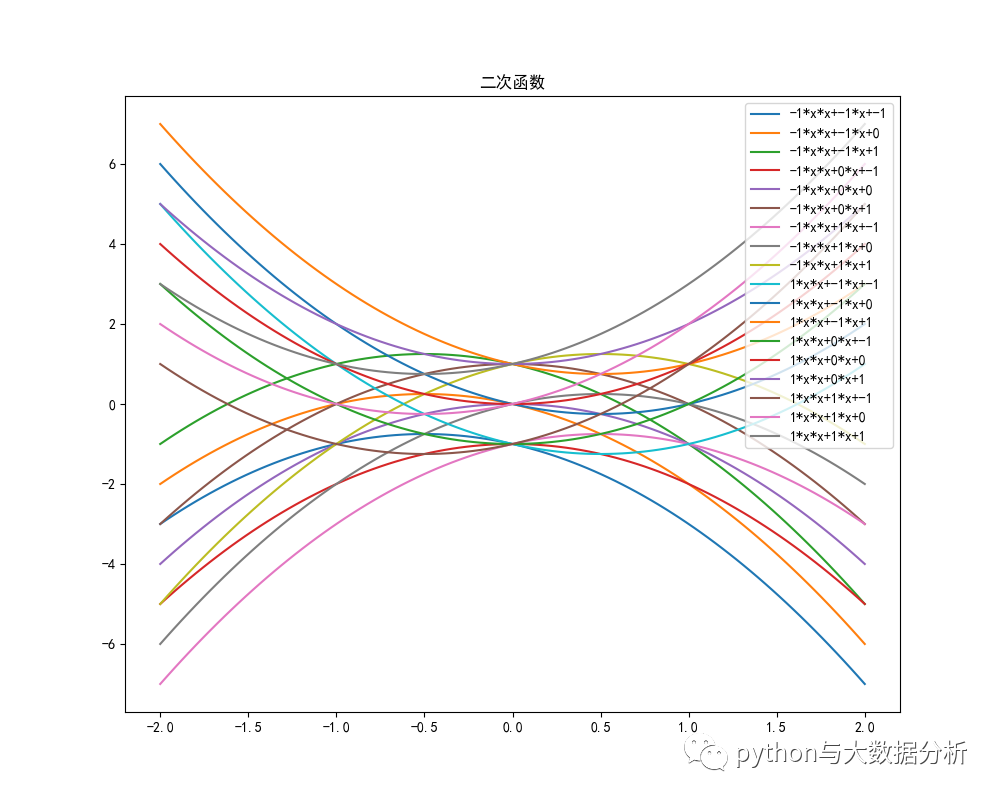幂函数代码如下：
# 幂函数是基本初等函数之一。
# 一般地，y=xα（α为有理数）的函数，即以底数为自变量，幂为因变量，指数为常数的函数称为幂函数。
# 例如函数y=x0 、y=x、y=x²、y=x³。
# a = 正数
# a 为>0 的自然数  x定义域(-∞,∞)
# a 为<0 的整数     X定义域(-∞,0),(0,∞)
#
# a >0 的分数
# a=n/m m为奇数，n为偶数，x定义域(-∞,∞)，y定义域[0,+∞）
# a=n/m m为奇数，n为奇数，x定义域(-∞,∞)，y定义域(-∞,∞)
# a=n/m m为偶数，n不限，x定义域[0,∞)，y定义域[0,+∞）
#
# a <0 的分数
# a=n/m m为奇数，n为偶数，x定义域(-∞,0),(0,∞)，y定义域(0,+∞）
# a=n/m m为奇数，n为奇数，x定义域(-∞,0),(0,∞)，y定义域(-∞,0),(0,∞)
# a=n/m m为偶数，n不限，x定义域(0,∞)，y定义域(0,+∞）
def powerfunction():
plt.figure(figsize=(10, 8))
ax = plt.gca()  # 通过gca:get current axis得到当前轴
plt.rcParams['font.sans-serif'] = ['SimHei']  # 绘图中文
plt.rcParams['axes.unicode_minus'] = False  # 绘图负号
x = np.linspace(-2, 2, 100)
alist = [1, 2, 3, 4]
for a in alist:
y = np.power(x, a)
label = 'math.pow(x,{}'.format(a)
plt.plot(x, y, label=label)

# 设置图片的右边框和上边框为不显示
ax.spines['right'].set_color('none')
ax.spines['top'].set_color('none')

# 挪动x，y轴的位置，也就是图片下边框和左边框的位置
# data表示通过值来设置x轴的位置，将x轴绑定在y=0的位置
ax.spines['bottom'].set_position(('data', 0))
# axes表示以百分比的形式设置轴的位置，即将y轴绑定在x轴50%的位置
# ax.spines['left'].set_position(('axes', 0.5))
ax.spines['left'].set_position(('data', 0))
plt.title("幂指数，a为正整数")
plt.legend()
plt.show()

plt.figure(figsize=(10, 8))
ax = plt.gca()  # 通过gca:get current axis得到当前轴
plt.rcParams['font.sans-serif'] = ['SimHei']  # 绘图中文
plt.rcParams['axes.unicode_minus'] = False  # 绘图负号
x = np.append(np.linspace(-1, -0.01, 100), np.linspace(0.01, 1, 100))
alist = [-1, -2, -3]
for a in alist:
y = np.power(x, a)
label = 'math.pow(x,{}'.format(a)
plt.plot(x, y, label=label)

# 设置图片的右边框和上边框为不显示
ax.spines['right'].set_color('none')
ax.spines['top'].set_color('none')

# 挪动x，y轴的位置，也就是图片下边框和左边框的位置
# data表示通过值来设置x轴的位置，将x轴绑定在y=0的位置
ax.spines['bottom'].set_position(('data', 0))
# axes表示以百分比的形式设置轴的位置，即将y轴绑定在x轴50%的位置
# ax.spines['left'].set_position(('axes', 0.5))
ax.spines['left'].set_position(('data', 0))
plt.title("幂指数，a为负整数")
plt.legend()
plt.show()

# a >0 的分数
# a=n/m m为奇数，n为奇数，x定义域(-∞,∞)，y定义域(-∞,∞) 4/3
# a=n/m m为奇数，n为偶数，x定义域[0,+∞)，y定义域[0,+∞）4/3
# a=n/m m为偶数，n不限，x定义域(-∞,∞)，y定义域[0,+∞） 1/2,3/2
plt.figure(figsize=(10, 8))
ax = plt.gca()  # 通过gca:get current axis得到当前轴
plt.rcParams['font.sans-serif'] = ['SimHei']  # 绘图中文
plt.rcParams['axes.unicode_minus'] = False  # 绘图负号
x = np.linspace(-2, 2, 100)
alist = [1 / 3, 5 / 3, 7 / 3]
for a in alist:
# y = np.power(x, a)
# RuntimeWarning: invalid value encountered in power
y = np.float_power(abs(x), a) * np.sign(x)
label = 'math.pow(x,{}'.format(a)
plt.plot(x, y, label=label)

# 设置图片的右边框和上边框为不显示
ax.spines['right'].set_color('none')
ax.spines['top'].set_color('none')

# 挪动x，y轴的位置，也就是图片下边框和左边框的位置
# data表示通过值来设置x轴的位置，将x轴绑定在y=0的位置
ax.spines['bottom'].set_position(('data', 0))
# axes表示以百分比的形式设置轴的位置，即将y轴绑定在x轴50%的位置
# ax.spines['left'].set_position(('axes', 0.5))
ax.spines['left'].set_position(('data', 0))
plt.title("幂指数，分子分母为奇数")
plt.legend()
plt.show()

plt.figure(figsize=(10, 8))
ax = plt.gca()  # 通过gca:get current axis得到当前轴
plt.rcParams['font.sans-serif'] = ['SimHei']  # 绘图中文
plt.rcParams['axes.unicode_minus'] = False  # 绘图负号
x = np.linspace(0, 2, 100)
alist = [1 / 8, 1 / 4, 1 / 2]
for a in alist:
y = np.power(x, a)
# y = np.float_power(abs(x), a) * np.sign(x)
label = 'math.pow(x,{}'.format(a)
plt.plot(x, y, label=label)

# 设置图片的右边框和上边框为不显示
ax.spines['right'].set_color('none')
ax.spines['top'].set_color('none')

# 挪动x，y轴的位置，也就是图片下边框和左边框的位置
# data表示通过值来设置x轴的位置，将x轴绑定在y=0的位置
ax.spines['bottom'].set_position(('data', 0))
# axes表示以百分比的形式设置轴的位置，即将y轴绑定在x轴50%的位置
# ax.spines['left'].set_position(('axes', 0.5))
ax.spines['left'].set_position(('data', 0))
plt.title("幂指数，分母为偶数")
plt.legend()
plt.show()

plt.figure(figsize=(10, 8))
ax = plt.gca()  # 通过gca:get current axis得到当前轴
plt.rcParams['font.sans-serif'] = ['SimHei']  # 绘图中文
plt.rcParams['axes.unicode_minus'] = False  # 绘图负号
x = np.linspace(-2, 2, 100)
alist = [2 / 3, 4 / 5, 6 / 7, 4 / 3, 8 / 5]
for a in alist:
y = np.power(abs(x), a)
label = 'math.pow(x,{}'.format(a)
plt.plot(x, y, label=label)

# 设置图片的右边框和上边框为不显示
ax.spines['right'].set_color('none')
ax.spines['top'].set_color('none')

# 挪动x，y轴的位置，也就是图片下边框和左边框的位置
# data表示通过值来设置x轴的位置，将x轴绑定在y=0的位置
ax.spines['bottom'].set_position(('data', 0))
# axes表示以百分比的形式设置轴的位置，即将y轴绑定在x轴50%的位置
# ax.spines['left'].set_position(('axes', 0.5))
ax.spines['left'].set_position(('data', 0))
plt.title("幂指数，分子为偶数分母为奇数")
plt.legend()
plt.show()

# 关于负数就不再重复叙述了
# a <0 的分数
# a=n/m m为奇数，x定义域(-∞,0),(0,∞)，y定义域(0,+∞）
# a=n/m m为偶数，x定义域(-∞,0),(0,∞)，y定义域(-∞,0),(0,∞)
# a=n/m m为偶数，n为不限，x定义域(0,∞)，y定义域(0,+∞）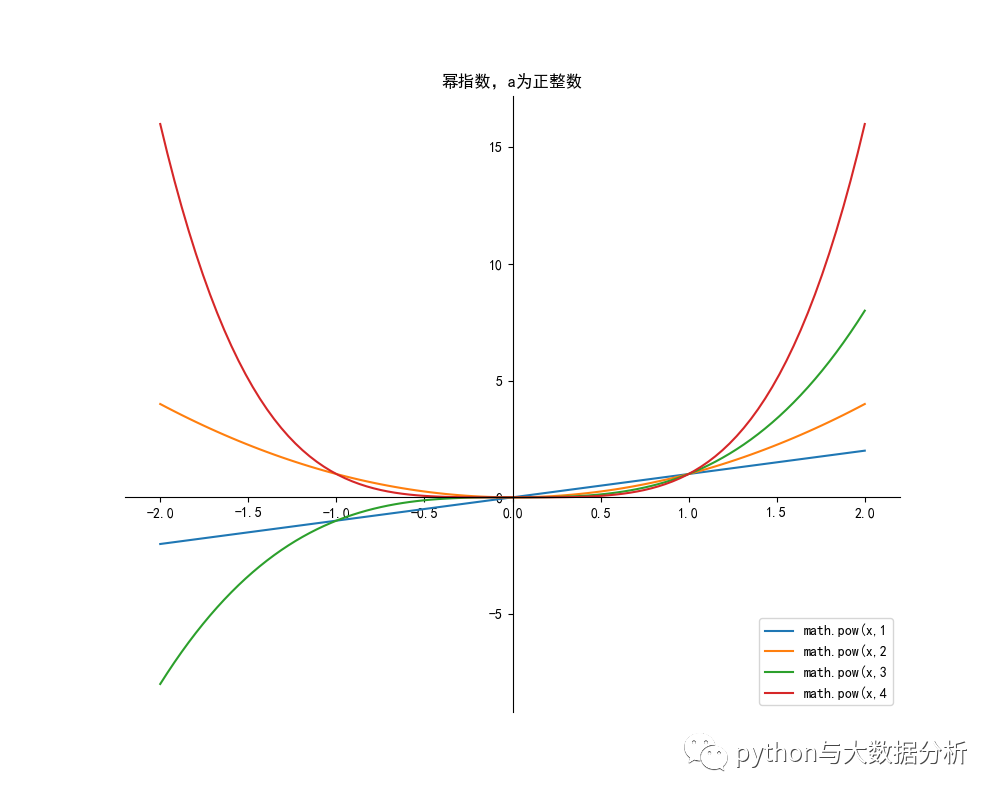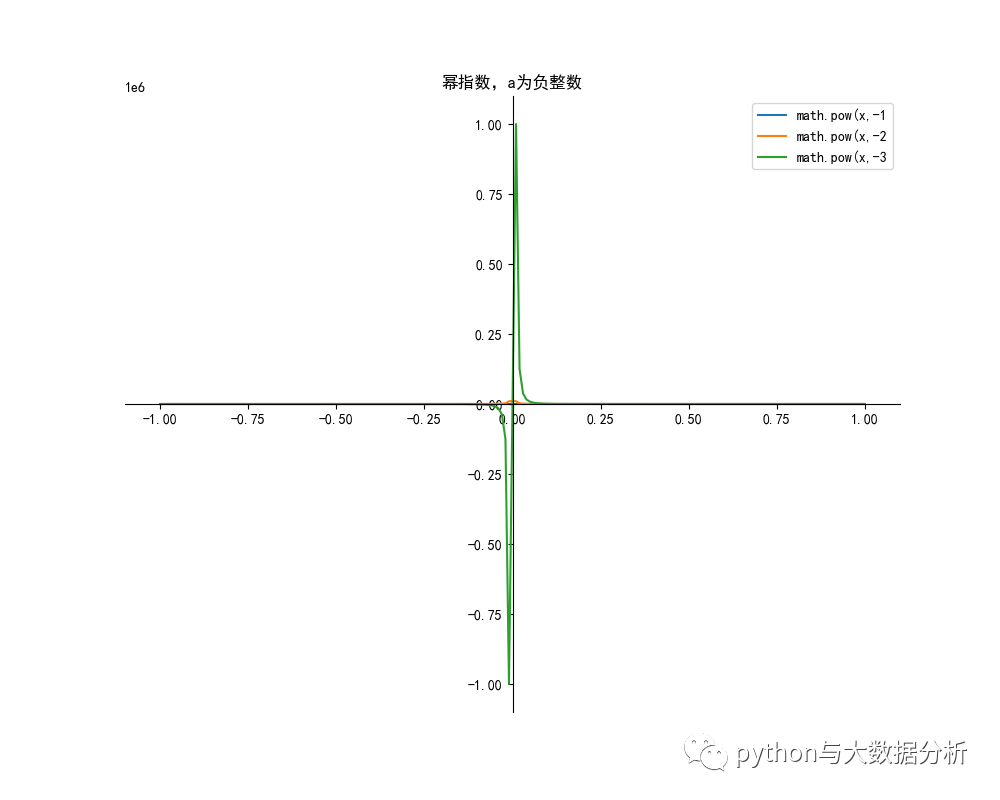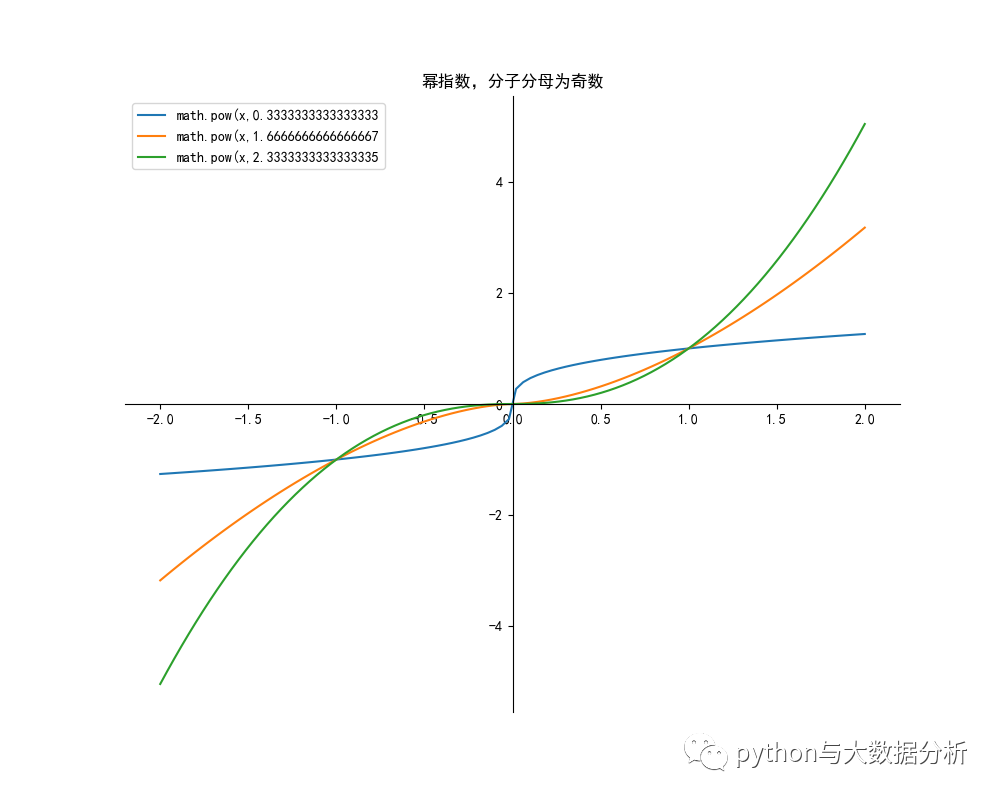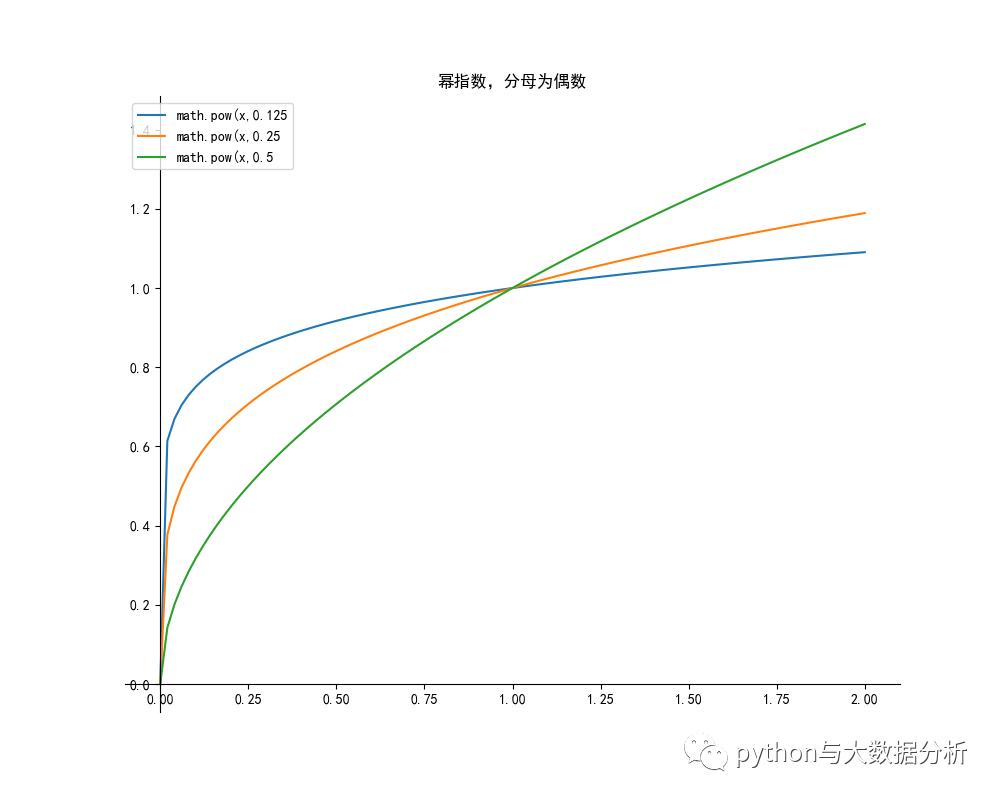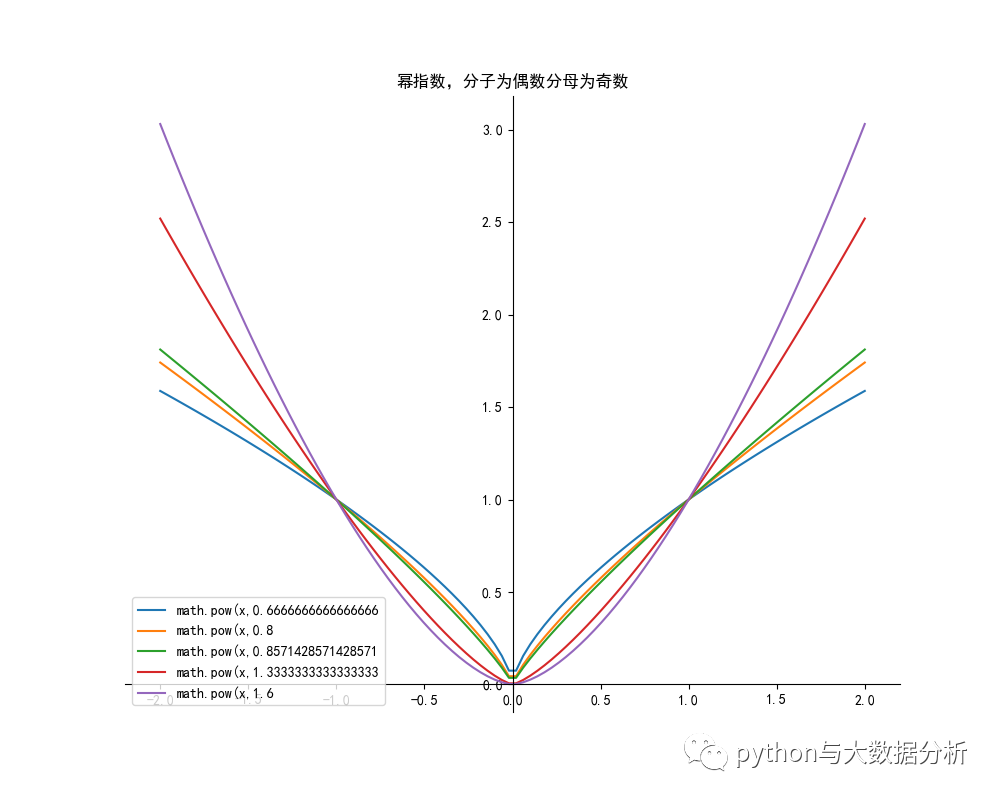指数函数代码如下：
# 指数函数是重要的基本初等函数之一。

# 一般地，y=ax函数(a为常数且以a>0，a≠1)叫做指数函数，函数的定义域是 R 。 
# 注意，在指数函数的定义表达式中，在ax前的系数必须是数1，自变量x必须在指数的位置上，且不能是x的其他表达式，
# 否则，就不是指数函数
def exponentialfunction():
plt.figure(figsize=(10, 8))
ax = plt.gca()  # 通过gca:get current axis得到当前轴
plt.rcParams['font.sans-serif'] = ['SimHei']  # 绘图中文
plt.rcParams['axes.unicode_minus'] = False  # 绘图负号
# a>0 a!=1
# 定义域为((-∞,∞)，值域为(0, +∞)，都通过(0, 1)点
x = np.linspace(-2, 2, 100)
alist = [1 / 4, 1 / 3, 1 / 2, 2, 3, 4]
for a in alist:
y = np.power(a, x)
label = 'math.pow(x,{}'.format(a)
plt.plot(x, y, label=label)

# 设置图片的右边框和上边框为不显示
ax.spines['right'].set_color('none')
ax.spines['top'].set_color('none')

# 挪动x，y轴的位置，也就是图片下边框和左边框的位置
# data表示通过值来设置x轴的位置，将x轴绑定在y=0的位置
ax.spines['bottom'].set_position(('data', 0))
# axes表示以百分比的形式设置轴的位置，即将y轴绑定在x轴50%的位置
# ax.spines['left'].set_position(('axes', 0.5))
ax.spines['left'].set_position(('data', 0))
plt.title("指数指数")
plt.legend()
plt.show()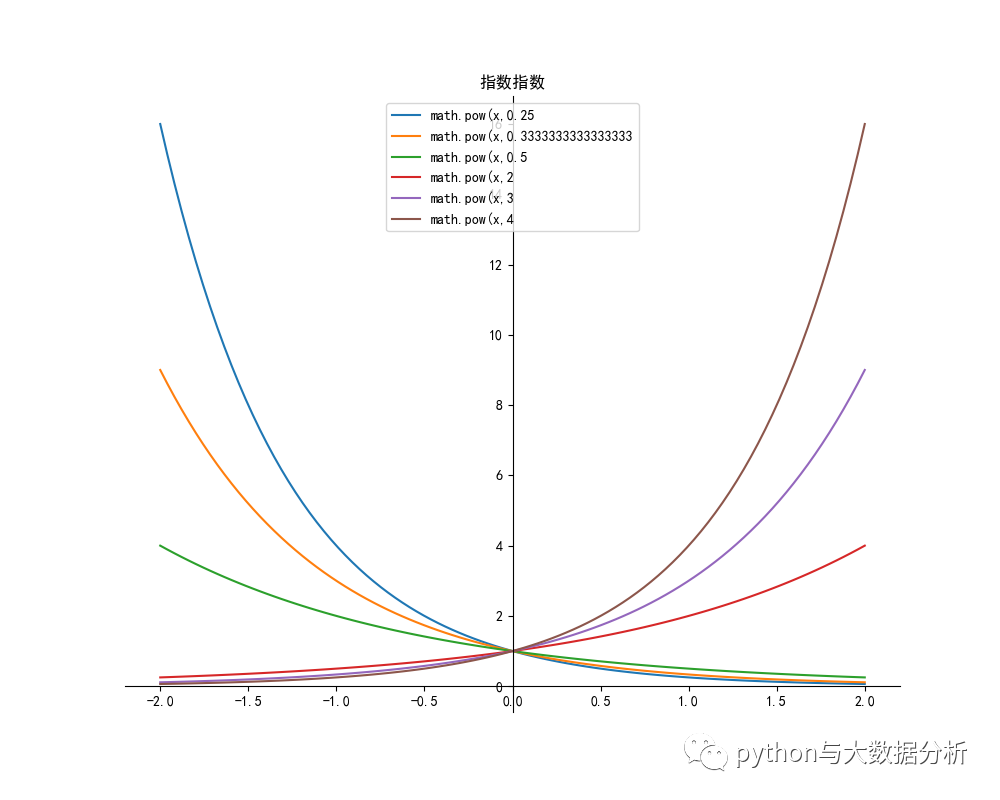对数函数代码如下：
# 一般地，对数函数是以幂（真数）为自变量，指数为因变量，底数为常量的函数。
# 对数函数是6类基本初等函数之一。其中对数的定义：
# 如果ax =N（a>0，且a≠1），那么数x叫做以a为底N的对数，记作x=logaN，读作以a为底N的对数，其中a叫做对数的底数，N叫做真数。
# 一般地，函数y=logaX（a>0，且a≠1）叫做对数函数，也就是说以幂（真数）为自变量，指数为因变量，底数为常量的函数，叫对数函数。
# 其中x是自变量，函数的定义域是（0，+∞），即x>0。它实际上就是指数函数的反函数，可表示为x=ay。因此指数函数里对于a的规定，同样适用于对数函数。
def Logarithmicfunction():
plt.figure(figsize=(10, 8))
ax = plt.gca()  # 通过gca:get current axis得到当前轴
plt.rcParams['font.sans-serif'] = ['SimHei']  # 绘图中文
plt.rcParams['axes.unicode_minus'] = False  # 绘图负号
# a>0 a!=1
# 定义域为((-∞,∞)，值域为(0, +∞)，都通过(0, 1)点
# 当a>1时，单调递增
# 当0<a<1时，单调递减
x = np.linspace(0.0001, 2, 100)
alist = [1 / 4, 1 / 3, 1 / 2, 2, 3, 4]
for a in alist:
y = np.log(x) / np.log(a)

      label = 'np.log(x) / np.log({})'.format(a)
      plt.plot(x, y, label=label)

# 设置图片的右边框和上边框为不显示
ax.spines['right'].set_color('none')
ax.spines['top'].set_color('none')

# 挪动x，y轴的位置，也就是图片下边框和左边框的位置
# data表示通过值来设置x轴的位置，将x轴绑定在y=0的位置
ax.spines['bottom'].set_position(('data', 0))
# axes表示以百分比的形式设置轴的位置，即将y轴绑定在x轴50%的位置
# ax.spines['left'].set_position(('axes', 0.5))
ax.spines['left'].set_position(('data', 0))
plt.title("对数指数")
plt.legend()
plt.show()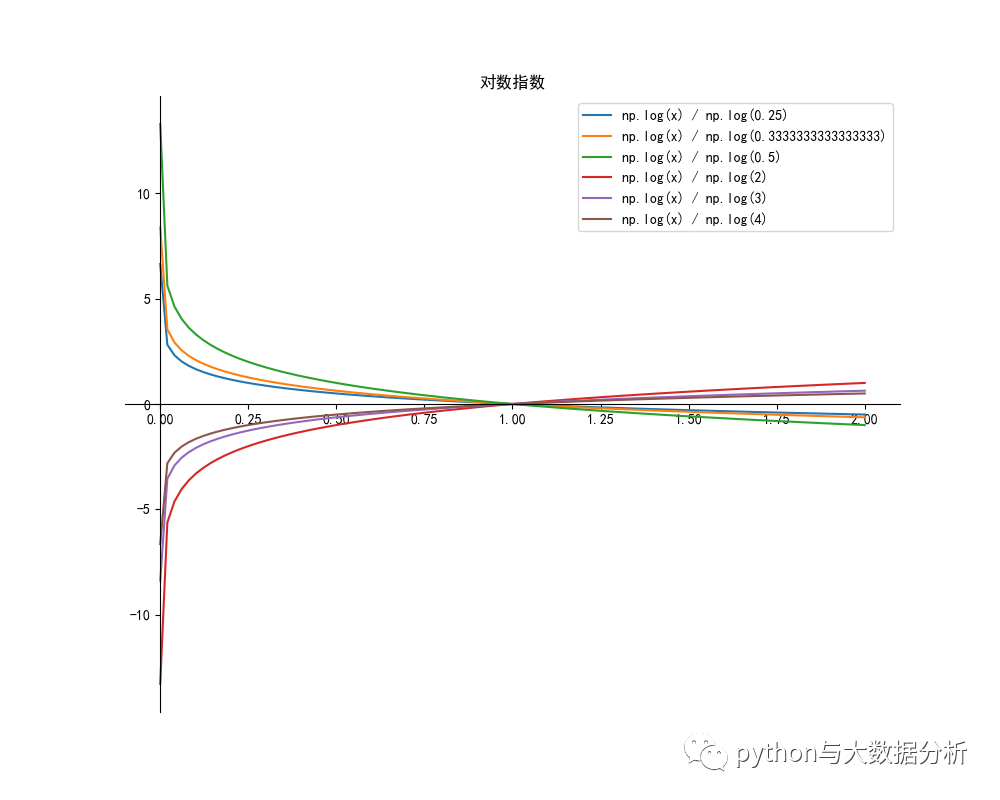展开全文graphviz ggplot2 可视化 wxpython less
• 存在反函数(默认为单值函数)的条件是原函数必须是一一对应的(不一定是整个数域内的)，这里的一一对应是定义域和值域的一一对应 注意：上标"−1"指的并不是幂, 代表反函数 最具有代表性的反函数就是对数函数与指数...
反函数
1 ) 概念
一般地，如果x与y关于某种对应关系f(x)相对应，y = f(x)，则y = f(x)的反函数为 x = f(y) 或者

y

=

f

−

1

(

x

)

y = f^{-1}(x)

后者为常用记发存在反函数(默认为单值函数)的条件是原函数必须是一一对应的(不一定是整个数域内的)，这里的一一对应是定义域和值域的一一对应注意：上标"−1"指的并不是幂, 代表反函数最具有代表性的反函数就是对数函数与指数函数，再比如:

y

=

x

3

y = x^3

和

y

=

x

3

y = \sqrt{x}

2 ) 性质
函数f(x)与它的反函数

y

=

f

−

1

(

x

)

y = f^{-1}(x)

图象关于直线y = x对称函数存在反函数的充要条件是，函数的定义域与值域是一一映射；一个函数与它的反函数在相应区间上单调性一致；大部分偶函数不存在反函数（当函数y = f(x)， 定义域是{0} 且 f(x) = C （其中C是常数），则函数f(x)是偶函数且有反函数，其反函数的定义域是{C}，值域为{0} ）。奇函数不一定存在反函数，被与y轴垂直的直线截时能过2个及以上点即没有反函数。若一个奇函数存在反函数，则它的反函数也是奇函数。备注：奇函数关于原点对称，偶函数关于y轴对称一段连续的函数的单调性在对应区间内具有一致性严增（减）的函数一定有严格增（减）的反函数反函数是相互的且具有唯一性定义域、值域相反对应法则互逆（三反）
六个基本初等函数
1 ) 分类
基本初等函数包括幂函数、指数函数、对数函数、三角函数、反三角函数和常数函数
2 ） 幂函数
一般地, 形如

y

=

x

a

y=x^a

(a为有理数)的函数，即以底数为自变量，幂为因变量，指数为常数的函数称为幂函数其中，a可为任何常数，但中学阶段仅研究a为有理数的情形a为无理数时取其近似的有理数, 例如函数

y

=

x

0

、

y

=

x

1

、

y

=

x

2

、

y

=

x

−

1

y = x^0 、y = x^1、y = x^2、y = x^{-1}

注：

y

=

x

−

1

=

1

x

y=x^{-1} = \frac{1}{x}

;

y

=

x

0

y=x^0

时, x≠0 等都是幂函数
3 ） 指数函数
一般地，函数

y

=

a

x

y=a^x

(a为常数且以a>0，a≠1)叫做指数函数，函数的定义域是R。对于一切指数函数来讲，值域为(0， +∞)。即：

y

=

a

x

(

a

>

0

且

a

≠

1

)

x

∈

R

y

∈

(

0

,

+

∞

)

y = a^x \ \ (a > 0 且 a \neq 1) \ \ x \in R \ \ \ y \in (0, +\infty)

指数函数中, 前面的系数为1。 如 :

y

=

1

0

x

、

y

=

π

x

y=10^x 、 y=\pi^x

都是指数函数； 而

y

=

2

∗

3

x

y = 2*3^x

不是指数函数
指数函数的4个运算法则

a

m

+

n

=

a

m

∗

a

n

a^{m+n} = a^m * a^n

a

m

n

=

(

a

m

)

n

a^{mn} = (a^m)^n

a

1

n

=

a

n

a^{\frac{1}{n}} = \sqrt[n]{a}

a

m

−

n

=

a

m

a

n

a^{m-n} = \frac{a^m}{a^n}

4 ） 对数函数
对数函数是指数函数的反函数

y

=

l

o

g

a

x

y = log_a x

，

x

∈

(

0

,

+

∞

)

x \in (0, + \infty)

,

y

∈

R

y \in R

，其中a为底数，a > 0 且 a ≠ 1，等价于

a

y

=

x

a^y = x

当 a > 1时，递增；当 0 < a < 1时，递减
常用对数运算

l

o

g

a

M

N

=

l

o

g

a

M

+

l

o

g

a

N

log_a {MN} = log_a M + log_a N

l

o

g

a

M

N

=

l

o

g

a

M

−

l

o

g

a

N

log_a {\frac{M}{N}} = log_a M - log_a N

l

o

g

a

a

b

=

b

log_a a^b = b

a

l

o

g

a

N

=

N

a^{log_a N} = N

l

o

g

1

0

b

=

l

g

b

log_10 b = lg b

l

o

g

e

b

=

l

n

b

log_e b = ln b

l

o

g

a

b

=

l

o

g

c

b

l

o

g

c

a

log_a b = \frac{log_c b}{log_c a}

换底公式

l

o

g

a

n

b

m

=

m

n

l

o

g

a

b

log_{a^n} b^m = \frac{m}{n} log_a b

指系公式

l

o

g

a

b

=

l

n

b

l

n

a

=

1

l

n

a

l

n

b

=

1

l

o

g

b

a

log_a b = \frac{ln b}{ln a} = \frac{1}{\frac{ln a}{ln b}} = \frac{1}{log_b a}

倒数公式

l

o

g

a

b

∗

l

o

g

c

a

=

l

n

b

l

n

a

∗

l

n

a

l

n

c

=

l

n

b

l

n

c

=

l

o

g

c

b

log_a b * log_c a = \frac{ln b}{ln a} * \frac{ln a}{ln c} = \frac{ln b}{ln c} = log_c b

链式公式
5 ） 三角函数
三角函数是基本初等函数之一是以角度（数学上最常用弧度制，下同）为自变量，角度对应任意角终边与单位圆交点坐标或其比值为因变量的函数。也可以等价地用与单位圆有关的各种线段的长度来定义。三角函数在研究三角形和圆等几何形状的性质时有重要作用，也是研究周期性现象的基础数学工具。在数学分析中，三角函数也被定义为无穷级数或特定微分方程的解，允许它们的取值扩展到任意实数值，甚至是复数值。常见的三角函数包括正弦函数、余弦函数和正切函数。在航海学、测绘学、工程学等其他学科中，还会用到如余切函数、正割函数、余割函数、正矢函数、余矢函数、半正矢函数、半余矢函数等其他的三角函数。不同的三角函数之间的关系可以通过几何直观或者计算得出，称为三角恒等式。在直角三角形ABC中，角A为90度，设角B为

θ

\theta

, 边BC记为a, 边AC记为b, 边AB记为c勾股定理：直角三角形中

a

2

=

b

2

+

c

2

a^2 = b^2 + c^2

s

i

n

θ

=

b

a

<

1

sin \theta = \frac{b}{a} < 1

正弦函数

c

o

s

θ

=

c

a

<

1

cos \theta = \frac{c}{a} < 1

余弦函数

t

a

n

θ

=

b

c

tan \theta = \frac{b}{c}

正切函数

c

o

t

θ

=

c

b

cot \theta = \frac{c}{b}

余切函数

s

e

c

θ

=

a

c

sec \theta = \frac{a}{c}

正割函数

c

s

c

θ

=

a

b

csc \theta = \frac{a}{b}

余割函数一般我们可以将三角形放入直角坐标系中来处理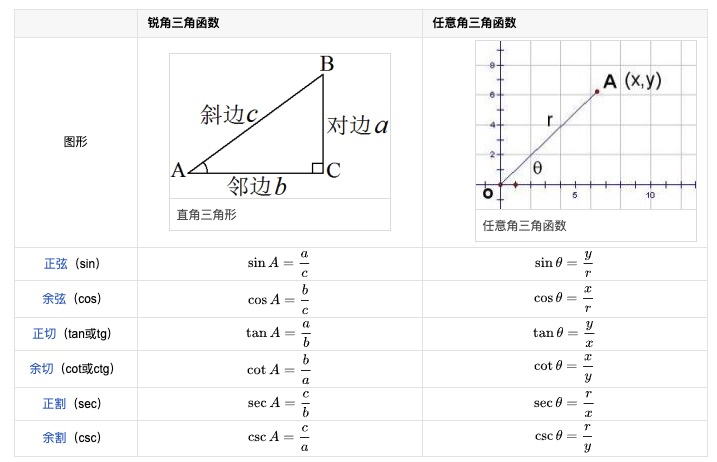备注：图片托管于github，请确保网络的可访问性

上图和我们的假设条件不一致，仅作为参考
以下为六种函数图像，均为周期函数，只展示一部分, 画图软件为Mac平台的Grapher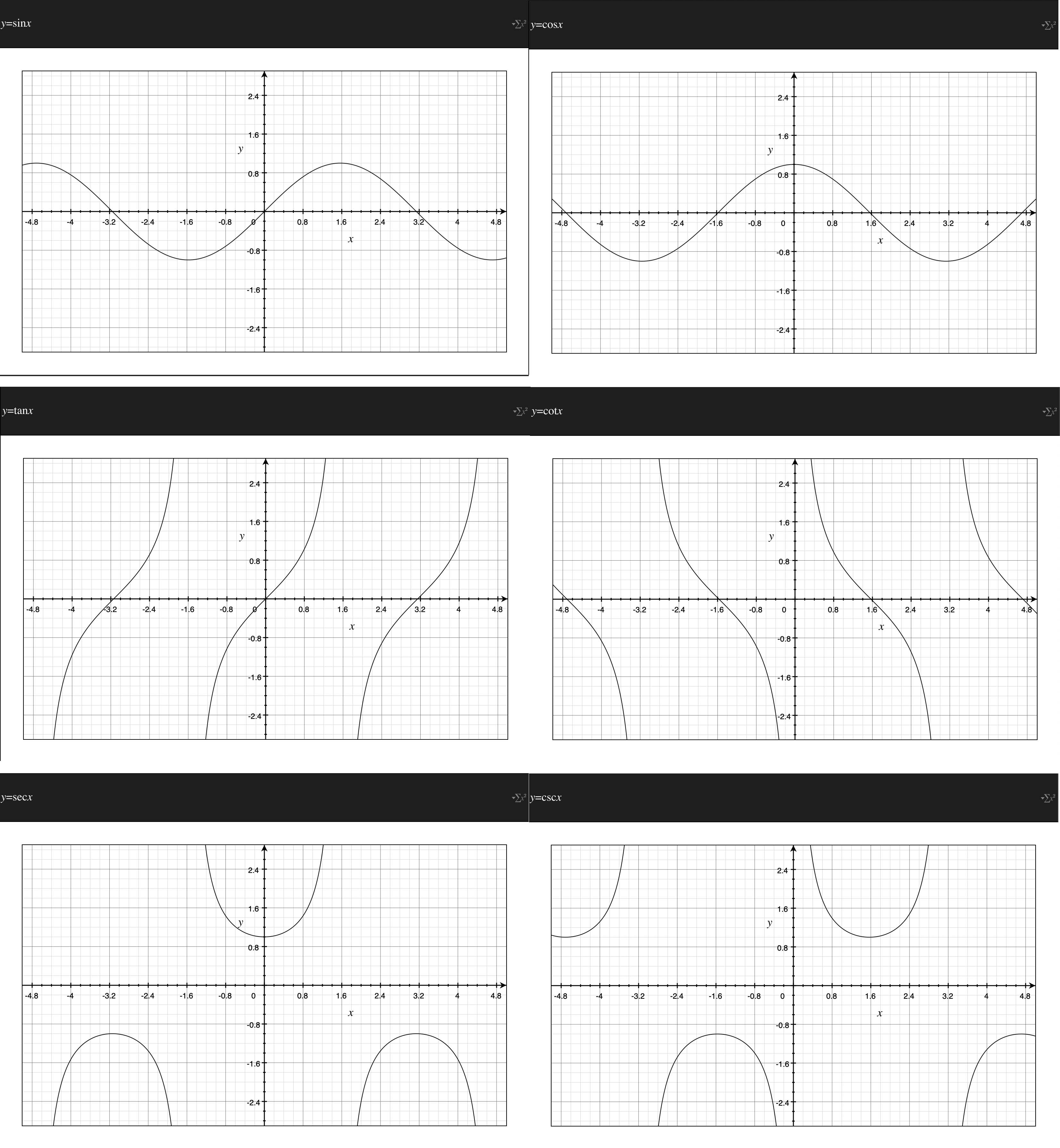备注：图片托管于github，请确保网络的可访问性

相关公式非常之多，不再这里赘述
6 ） 反三角函数
反三角函数是一种基本初等函数。它是反正弦arcsin x，反余弦arccos x，反正切arctan x，反余切arccot x，反正割arcsec x，反余割arccsc x这些函数的统称各自表示其反正弦、反余弦、反正切、反余切 ，反正割，反余割为x的角。三角函数的反函数是个多值函数，因为它并不满足一个自变量对应一个函数值的要求，其图像与其原函数关于函数 y=x 对称。欧拉提出反三角函数的概念，并且首先使用了“arc+函数名”的形式表示反三角函数。如果一个三角函数是：

y

=

s

i

n

x

y = sin x

, 则其反三角函数为：

y

=

a

r

c

s

i

n

x

y = arc sin x

因为不同的x可以对应同一个y值，属于多对一的现象，原则上是不存在反函数的我们在研究三角函数的反函数的时候可以设定一个区间，如[-π/2, π/2], 单调递增是有反函数的，值域范围在 [-1, 1]，即：y = sinx,

x

∈

[

−

π

2

,

π

2

]

x \in [-\frac{\pi}{2}, \frac{\pi}{2}]

y

∈

[

−

1

,

1

]

y \in [-1, 1]

单调递增则其反函数：y = arcsinx,

x

∈

[

−

1

,

1

]

x \in [-1, 1]

,

y

∈

[

−

π

2

,

π

2

]

y \in [-\frac{\pi}{2}, \frac{\pi}{2}]

7 ） 常数函数
在数学中，常数函数（也称常值函数）是指值不发生改变（即是常数）的函数。例如：y = 5常数函数都是偶函数`
展开全文AI 数学
• 基本初等函数分为幂函数、指数函数、对数函数、三角函数、反三角函数。 幂函数： 指数函数： 对数函数： 三角函数： 正弦函数： 余弦函数： 正切函数： 余切函数： 正割函数： 余割函数： 其它：正矢函数、...
• 重难点重点基本初等函数定义图象和性质由复PPT课件.pptx
•python
• ## 初等函数公式汇总

万次阅读 2018-09-18 10:27:23
函数函数的一般形式为y=x^a. 　如果a取非零的有理数是比较容易理解的,不过初学者对于a取无理数,则不太容易理解,在我们的课程里,不要求掌握如何理解指数为无理数的问题,因为这涉及到实数连续统的极为深刻的知识....
• 关于初等函数，《高等数学》（同济七版）中是这样子定义的：由常数和基本初等函数经过有限次的四则运算和有限次的函数复合步骤所构成并可用一个式子表示的函数，称为初等函数。 这个定义中，有两个需要注意的：(1) ...
• 内容来自百度百科知识以及东方耀老师笔记内容的整合 1、反函数 1.1 定义 ...一般地，设函数y=f(x)(x∈A)的值域是C，...反函数y=f ^(-1)(x)的定义域、值域分别是函数y=f(x)的值域、定义域。最具有代表性的反函数就是
• 初等函数（基本函数）是由常函数、幂函数、指数函数、对数函数、三角函数和反三角函数经过有限次的有理运算（加、减、乘、除、有限次乘方、有限次开方）及有限次函数复合所产生、并且在定义域上能用一个方程式表示的...
• 2019高考数学一轮复习第2章函数与基本初等函数第2课时函数的定义域与值域练习理
• 连续函数的运算 （一）四则运算 定理1：设f(x),g(x)在x=x0处连续，则 ①f(x)±g(x)在x=x0处连续; ②f(x)g(x)在x=x0处连续...回顾：初等函数是由常数和基本初等函数经过四则和复合运算形成的函数 直接给结论: ①基本初等
• 定义域和定义区间，自然不是一样的。 1：定义域就是定义域，函数三特性之一。但是其表现形式不见得非要是区间...1：基本初等函数定义域内连续 2：初等函数定义区间内连续 比如这么一个函数f(x)=1他定义域是x=...
• 数学里的六类基本初等函数，我们已经介绍了指数函数和对数函数，还剩常数函数，幂函数，三角函数和反三角函数，这一期，我们重点介绍后面四类基本初等函数。常数函数一般的，形如的函数称为常数函数，...
• 文章目录两个重要极限初等函数的导数反函数的导数复合函数的导数泰勒展开罗尔中值定理拉格朗日中值定理柯西中值定理洛必达法则泰勒展开 两个重要极限 limn→∞(1+1n)=elim_{n \to \infin}(1+{1\over n}) = elimn→...
• §1.10 连续函数的运算与初等函数的连续性 一、连续函数的四则运算性质 由函数在一点连续的定义，不难发现，函数连续的问题仍是一个函数的极限问题，而函数极限的四则运算法则业已证明，因此，我们只要稍加改动，...高等数学
• 一般来说，设函数y=f(x)(x∈A)的值域是C，若找得到一个函数g(y)在每一处g(y)都等于x，这样的函数x= g(y)(y∈C)叫做函数y=f(x)(x∈A)的反函数，记作x=f-1...qt 数据可视化 html 可视化 less
• 定义域条件 分式中的分母不为零 偶次方根内大于等于0 2x−12x−1>=0 \sqrt{2x-1} \qquad 2x-1>=0 2x−1​2x−1>=0 对数真数大于0 如果ax =N（a>0，且a≠1），那么数x叫做以a为底N的对数，记作x=logaN...
• 2019_2020学年高中数学第1章基本初等函数Ⅱ1.2.1三角函数的定义练习新人教B版必修4
• 欢迎点击「算法与编程之美」↑关注我们！本文首发于微信公众号："算法与编程之美"，欢迎关注，及时了解更多此系列博客。在上一篇文章数学分析将基本初等函数归为六类：幂函数、指数...
• 由常数和基本初等函数经过有限次的四则运算和有限次的函数复合步骤所构成并可用一个式子表示的函数，称为初等函数。 二 幂函数 y=x^α（α为有理数且为常数）的函数，即以底数为自变量，幂为因变量，指数为常数的...数学
• 一、反函数 1.1、反函数的定义与性质 二、复合函数 三、初等函数初等函数 实在不能通过函数来表达，使用分段函数来表示 小结
• 欢迎点击「算法与编程之美」↑关注我们！本文首发于微信公众号："算法与编程之美"，欢迎关注，及时了解更多此系列博客。本文为大家介绍对数函数定义一般地，函数y=logax...
• 常数和基本初等函数导数公式： 1. c ′ = 0 c' = 0 2. ( x n ) ′ = n x n − 1 (x^n)' = nx^{n-1} (1). ( x √ ) ′ = 1 2 x √ (\sqrt x)' = \frac{1}{2\sqrt x} (2). ( 1 x ) ′ = − 1 x 2 (\...数学 x...